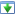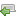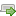Choose a version here. If you've not already started the tutorial, just go with the latest one.

 30 Aug 2014 Preview version Choose 5 Oct 2014 Explicitly adds a specific charset for htmlspecialchars(), and wraps it with a custom function Choose 16 Oct 2014 Updated redirect function to work with vhost subfolders Choose 4 Nov 2014 Minor improvements: added missing docblock, fixed security issue, CSS tweak. Switched hashing method to DEFAULT instead of BCRYPT, this is best practice. Choose 25 Nov 2014 Improve the notes on getting started, in particular choosing a programmer's editor. Added introduction to mod_rewrite rules. Choose ♥ 16 Aug 2018 Some bug fixes, remove compatibility library for earlier version of PHP Choose
OK
NB: There are several versions of this tutorial, each successive one containing additional improvements. If you're in the middle of working through it, please check the versions panel above, to ensure you're not mixing code from different versions.

## New post creation

Let's turn our attention to another major feature: writing a new article. We start off with setting up a new page, laying out the necessary form input controls, and adding a new logged-in menu item. There's also a change here to add a generic class `user-form`, so our forms across the whole application can easily acquire a common look-and-feel; see how I've gone back to existing form comment-form.php to update that too.`82`
`83`
`84`
`85`
`86`
`87`
`88`
`89`
`90`
`91`
`92`
`93`
` margin-bottom: 8px;`
`}`
`.comment-form input,`
`.comment-form textarea {`
` margin: 4px;`
`}`
`.comment-form label {`
` font-size: 0.95em;`
` margin: 6px;`
` width: 7em;`
`82`
`83`
`84`
`85`
`86`
`87`
`88`
`89`
`90`
`91`
`92`
`93`
` margin-bottom: 8px;`
`}`
`.user-form input,`
`.user-form textarea {`
` margin: 4px;`
`}`
`.user-form label {`
` font-size: 0.95em;`
` margin: 6px;`
` width: 7em;`
`1`
`2`
`3`
`4`
`5`
`6`
`7`
`8`
`9`
`10`
`11`
`12`
`13`
`14`
`15`
`16`
`17`
`18`
`19`
`20`
`21`
`22`
`23`
`24`
`25`
`26`
`27`
`28`
`29`
`30`
`31`
`32`
`33`
`34`
`35`
`36`
`37`
`38`
`39`
`40`
`41`
`42`
`<?php`
`require_once 'lib/common.php';`
`session_start();`
`?>`
`<!DOCTYPE html>`
`<html>`
` <head>`
` <title>A blog application | New post</title>`
` <?php require 'templates/head.php' ?>`
` </head>`
` <body>`
` <?php require 'templates/title.php' ?>`
` <form method="post" class="post-form user-form">`
` <div>`
` <label for="post-title">Title:</label>`
` <input`
` id="post-title"`
` name="post-title"`
` type="text"`
` />`
` </div>`
` <div>`
` <label for="post-body">Body:</label>`
` <textarea`
` id="post-body"`
` name="post-body"`
` rows="12"`
` cols="70"`
` ></textarea>`
` </div>`
` <div>`
` <input`
` type="submit"`
` value="Save post"`
` />`
` </div>`
` </form>`
` </body>`
`</html>`
`18`
`19`
`20`
`21`
`22`
`23`
`24`
`<h3>Add your comment</h3>`
`<form method="post" class="comment-form">`
` <div>`
` <label for="comment-name">`
` Name:`
`18`
`19`
`20`
`21`
`22`
`23`
`24`
`<h3>Add your comment</h3>`
`<form method="post" class="comment-form user-form">`
` <div>`
` <label for="comment-name">`
` Name:`
`1`
`2`
`3`
` `
` `
`4`
`5`
`6`
`<div class="top-menu">`
` <div class="menu-options">`
` <?php if (isLoggedIn()): ?>`
` Hello <?php echo htmlEscape(getAuthUser()) ?>.`
` <a href="logout.php">Log out</a>`
` <?php else: ?>`
`1`
`2`
`3`
`4`
`5`
`6`
`7`
`8`
`<div class="top-menu">`
` <div class="menu-options">`
` <?php if (isLoggedIn()): ?>`
` <a href="edit-post.php">New post</a>`
` |`
` Hello <?php echo htmlEscape(getAuthUser()) ?>.`
` <a href="logout.php">Log out</a>`
` <?php else: ?>`

Now, we only want to show this page for users who are logged in. Thus, if a user who is not logged in tries to access it (by typing the URL in directly) we must redirect them elsewhere; in this case, the home page will do fine.`3`
`4`
`5`
` `
` `
` `
` `
` `
` `
`6`
`7`
`8`
`session_start();`
`?>`
`<!DOCTYPE html>`
`<html>`
`3`
`4`
`5`
`6`
`7`
`8`
`9`
`10`
`11`
`12`
`13`
`14`
`session_start();`
`// Don't let non-auth users see this screen`
`if (!isLoggedIn())`
`{`
` redirectAndExit('index.php');`
`}`
`?>`
`<!DOCTYPE html>`
`<html>`

While we are here, let's take a look at how `redirectAndExit()` works; this is in common.php. It starts by reading the domain we are running on (such as `localhost`), since it is good not to hardwire this into our code. It then sends a `Location` HTTP header to the browser, which will cause it to request the specified address. Since the PHP script would happily carry on running at this point (until it has detected that the browser has disconnected) we also need to forcibly exit and wait for the redirect.

Although we now have the structure of the new post feature, it does not work yet. So let us fix that now:`1`
`2`
` `
`3`
`4`
`5`
`10`
`11`
`12`
` `
` `
` `
` `
` `
` `
` `
` `
` `
` `
` `
` `
` `
` `
` `
` `
` `
` `
` `
` `
` `
` `
` `
` `
` `
` `
` `
` `
` `
` `
` `
` `
` `
` `
` `
` `
` `
` `
` `
`13`
`14`
`15`
`59`
`60`
`61`
` `
` `
` `
` `
` `
` `
` `
` `
` `
` `
`62`
`63`
`64`
`<?php`
`require_once 'lib/common.php';`
`session_start();`
` redirectAndExit('index.php');`
`}`
`?>`
`<!DOCTYPE html>`
`<html>`
` <body>`
` <?php require 'templates/title.php' ?>`
` <form method="post" class="post-form user-form">`
` <div>`
` <label for="post-title">Title:</label>`
`1`
`2`
`3`
`4`
`5`
`6`
`10`
`11`
`12`
`13`
`14`
`15`
`16`
`17`
`18`
`19`
`20`
`21`
`22`
`23`
`24`
`25`
`26`
`27`
`28`
`29`
`30`
`31`
`32`
`33`
`34`
`35`
`36`
`37`
`38`
`39`
`40`
`41`
`42`
`43`
`44`
`45`
`46`
`47`
`48`
`49`
`50`
`51`
`52`
`53`
`54`
`59`
`60`
`61`
`62`
`63`
`64`
`65`
`66`
`67`
`68`
`69`
`70`
`71`
`72`
`73`
`74`
`<?php`
`require_once 'lib/common.php';`
`require_once 'lib/edit-post.php';`
`session_start();`
` redirectAndExit('index.php');`
`}`
`// Handle the post operation here`
`\$errors = array();`
`if (\$_POST)`
`{`
` // Validate these first`
` \$title = \$_POST['post-title'];`
` if (!\$title)`
` {`
` \$errors[] = 'The post must have a title';`
` }`
` \$body = \$_POST['post-body'];`
` if (!\$body)`
` {`
` \$errors[] = 'The post must have a body';`
` }`
` if (!\$errors)`
` {`
` \$pdo = getPDO();`
` \$userId = getAuthUserId(\$pdo);`
` \$postId = addPost(`
` getPDO(),`
` \$title,`
` \$body,`
` \$userId`
` );`
` if (\$postId === false)`
` {`
` \$errors[] = 'Post operation failed';`
` }`
` }`
` if (!\$errors)`
` {`
` redirectAndExit('edit-post.php?post_id=' . \$postId);`
` }`
`}`
`?>`
`<!DOCTYPE html>`
`<html>`
` <body>`
` <?php require 'templates/title.php' ?>`
` <?php if (\$errors): ?>`
` <div class="error box">`
` <ul>`
` <?php foreach (\$errors as \$error): ?>`
` <li><?php echo \$error ?></li>`
` <?php endforeach ?>`
` </ul>`
` </div>`
` <?php endif ?>`
` <form method="post" class="post-form user-form">`
` <div>`
` <label for="post-title">Title:</label>`
`205`
`206`
`207`
`{`
` return isset(\$_SESSION['logged_in_username']);`
`}`
`205`
`206`
`207`
`208`
`209`
`210`
`211`
`212`
`213`
`214`
`215`
`216`
`217`
`218`
`219`
`220`
`221`
`222`
`223`
`224`
`225`
`226`
`227`
`228`
`229`
`230`
`231`
`232`
`233`
`234`
`235`
`236`
`{`
` return isset(\$_SESSION['logged_in_username']);`
`}`
`/**`
` * Looks up the user_id for the current auth user`
` */`
`function getAuthUserId(PDO \$pdo)`
`{`
` // Reply with null if there is no logged-in user`
` if (!isLoggedIn())`
` {`
` return null;`
` }`
` \$sql = "`
` SELECT`
` id`
` FROM`
` user`
` WHERE`
` username = :username`
` ";`
` \$stmt = \$pdo->prepare(\$sql);`
` \$stmt->execute(`
` array(`
` 'username' => getAuthUser()`
` )`
` );`
` return \$stmt->fetchColumn();`
`}`
`1`
`2`
`3`
`4`
`5`
`6`
`7`
`8`
`9`
`10`
`11`
`12`
`13`
`14`
`15`
`16`
`17`
`18`
`19`
`20`
`21`
`22`
`23`
`24`
`25`
`26`
`27`
`28`
`29`
`30`
`31`
`32`
`33`
`34`
`<?php`
`function addPost(PDO \$pdo, \$title, \$body, \$userId)`
`{`
` // Prepare the insert query`
` \$sql = "`
` INSERT INTO`
` post`
` (title, body, user_id, created_at)`
` VALUES`
` (:title, :body, :user_id, :created_at)`
` ";`
` \$stmt = \$pdo->prepare(\$sql);`
` if (\$stmt === false)`
` {`
` throw new Exception('Could not prepare post insert query');`
` }`
` // Now run the query, with these parameters`
` \$result = \$stmt->execute(`
` array(`
` 'title' => \$title,`
` 'body' => \$body,`
` 'user_id' => \$userId,`
` 'created_at' => getSqlDateForNow(),`
` )`
` );`
` if (\$result === false)`
` {`
` throw new Exception('Could not run post insert query');`
` }`
` return \$pdo->lastInsertId();`
`}`

In a similar way to saving comments, we first test if we are in a post operation, using `if (\$_POST)`. This contains an array of input values that will be only be present if a user has submitted the form.

We then make some simple checks to ensure the form data is acceptable prior to our attempting to insert it into the database. This is a process known as form validation and is a frequent task within web application development. If any checks fail, we allow the page to be rendered in the POST request itself, plus error messages as appropriate, and it is only if the checks succeed that we try to save the data and redirect back to the newly committed article.

However, as it stands this redirect will just display a new, empty post, since we have not added the logic to render the edit facility for a specific row. Let's add that now:`1`
`2`
`3`
` `
`4`
`5`
`6`
`11`
`12`
`13`
` `
` `
` `
` `
` `
` `
`14`
`15`
`16`
`38`
`39`
`40`
`41`
`42`
`43`
`44`
`55`
`56`
`57`
` `
` `
` `
` `
` `
` `
` `
` `
` `
`58`
`59`
`60`
`92`
`93`
`94`
` `
`95`
`96`
`97`
`102`
`103`
`104`
`105`
`106`
`107`
`108`
`<?php`
`require_once 'lib/common.php';`
`require_once 'lib/edit-post.php';`
`session_start();`
` redirectAndExit('index.php');`
`}`
`// Handle the post operation here`
`\$errors = array();`
`if (\$_POST)`
` \$pdo = getPDO();`
` \$userId = getAuthUserId(\$pdo);`
` \$postId = addPost(`
` getPDO(),`
` \$title,`
` \$body,`
` \$userId`
` redirectAndExit('edit-post.php?post_id=' . \$postId);`
` }`
`}`
`?>`
`<!DOCTYPE html>`
` id="post-title"`
` name="post-title"`
` type="text"`
` />`
` </div>`
` <div>`
` name="post-body"`
` rows="12"`
` cols="70"`
` ></textarea>`
` </div>`
` <div>`
` <input`
`1`
`2`
`3`
`4`
`5`
`6`
`7`
`11`
`12`
`13`
`14`
`15`
`16`
`17`
`18`
`19`
`20`
`21`
`22`
`38`
`39`
`40`
`41`
`42`
`43`
`44`
`55`
`56`
`57`
`58`
`59`
`60`
`61`
`62`
`63`
`64`
`65`
`66`
`67`
`68`
`69`
`92`
`93`
`94`
`95`
`96`
`97`
`98`
`102`
`103`
`104`
`105`
`106`
`107`
`108`
`<?php`
`require_once 'lib/common.php';`
`require_once 'lib/edit-post.php';`
`require_once 'lib/view-post.php';`
`session_start();`
` redirectAndExit('index.php');`
`}`
`// Empty defaults`
`\$title = \$body = '';`
`// Init database and get handle`
`\$pdo = getPDO();`
`// Handle the post operation here`
`\$errors = array();`
`if (\$_POST)`
` \$pdo = getPDO();`
` \$userId = getAuthUserId(\$pdo);`
` \$postId = addPost(`
` \$pdo,`
` \$title,`
` \$body,`
` \$userId`
` redirectAndExit('edit-post.php?post_id=' . \$postId);`
` }`
`}`
`elseif (isset(\$_GET['post_id']))`
`{`
` \$post = getPostRow(\$pdo, \$_GET['post_id']);`
` if (\$post)`
` {`
` \$title = \$post['title'];`
` \$body = \$post['body'];`
` }`
`}`
`?>`
`<!DOCTYPE html>`
` id="post-title"`
` name="post-title"`
` type="text"`
` value="<?php echo htmlEscape(\$title) ?>"`
` />`
` </div>`
` <div>`
` name="post-body"`
` rows="12"`
` cols="70"`
` ><?php echo htmlEscape(\$body) ?></textarea>`
` </div>`
` <div>`
` <input`

That was nice and easy: if we find we are not in a post operation and we have a post primary key and that row exists, then show it in the edit form. You can see that now we need the database connection (in `\$pdo`) for two things, I've moved that line so that it is always executed.

As we have done before, this code uses our `htmlEscape()` function to prevent users from entering HTML, which could break our page layout or introduce security problems. It is perhaps less of a worry here, since at least these users are authenticated, and hence they might be considered more trustworthy than anonymous commenters.

If you tried editing a post, you'll have found that this created a new post, rather than updating the old one. So let's fix that also:`17`
`18`
`19`
` `
` `
` `
` `
` `
` `
` `
` `
` `
` `
` `
` `
`20`
`21`
`22`
`48`
`49`
`50`
`51`
`52`
`53`
`54`
`55`
`56`
`57`
`58`
`59`
`60`
`61`
` `
` `
` `
` `
` `
` `
` `
` `
` `
` `
`62`
`63`
`64`
`70`
`71`
`72`
`73`
`74`
`75`
`76`
`77`
`78`
`79`
`80`
`81`
`82`
`83`
`84`
`// Init database and get handle`
`\$pdo = getPDO();`
`// Handle the post operation here`
`\$errors = array();`
`if (\$_POST)`
` if (!\$errors)`
` {`
` \$pdo = getPDO();`
` \$userId = getAuthUserId(\$pdo);`
` \$postId = addPost(`
` \$pdo,`
` \$title,`
` \$body,`
` \$userId`
` );`
` if (\$postId === false)`
` {`
` \$errors[] = 'Post operation failed';`
` }`
` }`
` redirectAndExit('edit-post.php?post_id=' . \$postId);`
` }`
`}`
`elseif (isset(\$_GET['post_id']))`
`{`
` \$post = getPostRow(\$pdo, \$_GET['post_id']);`
` if (\$post)`
` {`
` \$title = \$post['title'];`
` \$body = \$post['body'];`
` }`
`}`
`?>`
`<!DOCTYPE html>`
`17`
`18`
`19`
`20`
`21`
`22`
`23`
`24`
`25`
`26`
`27`
`28`
`29`
`30`
`31`
`32`
`33`
`34`
`48`
`49`
`50`
`51`
`52`
` `
` `
` `
` `
` `
` `
` `
`53`
`54`
`55`
`56`
`57`
`58`
`59`
`60`
`61`
`62`
`63`
`64`
`65`
`66`
`67`
`70`
`71`
`72`
` `
` `
` `
` `
` `
` `
` `
` `
` `
`73`
`74`
`75`
`// Init database and get handle`
`\$pdo = getPDO();`
`\$postId = null;`
`if (isset(\$_GET['post_id']))`
`{`
` \$post = getPostRow(\$pdo, \$_GET['post_id']);`
` if (\$post)`
` {`
` \$postId = \$_GET['post_id'];`
` \$title = \$post['title'];`
` \$body = \$post['body'];`
` }`
`}`
`// Handle the post operation here`
`\$errors = array();`
`if (\$_POST)`
` if (!\$errors)`
` {`
` \$pdo = getPDO();`
` // Decide if we are editing or adding`
` if (\$postId)`
` {`
` editPost(\$pdo, \$title, \$body, \$postId);`
` }`
` else`
` {`
` \$userId = getAuthUserId(\$pdo);`
` \$postId = addPost(\$pdo, \$title, \$body, \$userId);`
` if (\$postId === false)`
` {`
` \$errors[] = 'Post operation failed';`
` }`
` }`
` }`
` redirectAndExit('edit-post.php?post_id=' . \$postId);`
` }`
`}`
`?>`
`<!DOCTYPE html>`
`32`
`33`
`34`
` return \$pdo->lastInsertId();`
`}`
`32`
`33`
`34`
`35`
`36`
`37`
`38`
`39`
`40`
`41`
`42`
`43`
`44`
`45`
`46`
`47`
`48`
`49`
`50`
`51`
`52`
`53`
`54`
`55`
`56`
`57`
`58`
`59`
`60`
`61`
`62`
`63`
`64`
`65`
`66`
`67`
`68`
` return \$pdo->lastInsertId();`
`}`
`function editPost(PDO \$pdo, \$title, \$body, \$postId)`
`{`
` // Prepare the insert query`
` \$sql = "`
` UPDATE`
` post`
` SET`
` title = :title,`
` body = :body`
` WHERE`
` id = :post_id`
` ";`
` \$stmt = \$pdo->prepare(\$sql);`
` if (\$stmt === false)`
` {`
` throw new Exception('Could not prepare post update query');`
` }`
` // Now run the query, with these parameters`
` \$result = \$stmt->execute(`
` array(`
` 'title' => \$title,`
` 'body' => \$body,`
` 'post_id' => \$postId,`
` )`
` );`
` if (\$result === false)`
` {`
` throw new Exception('Could not run post update query');`
` }`
` return true;`
`}`

I've moved the code to read the current post towards the start of the page, since this is now useful in two situations. The first is when displaying an article for editing, and the second is when submitting the edit form to save any changes. In both cases, `\$_GET['post_id']` will be available, and we can look up that row from the post table, and obtain title/body data if it is read successfully.

Within a POST operation, we can then check `\$postId`, and if it has a value we know we are editing an existing article rather than creating a new one. Thus, if we are editing, we call the new function `editPost()`, which will run the necessary `UPDATE` command against the database, rather than `addPost()`, which would run an `INSERT`.

You might have noticed that there has been no attempt to check whether the user editing a post is the same as the user who wrote it. Whether one person may edit other person's posts is a feature decision, but for the time being it is one that I have deliberately omitted, to keep things simple.